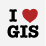# How to Calculate area in square meters from cell count

19558
6
04-05-2014 03:18 PMNew Contributor
I am using a cell size of 10 meters and I am trying to calculate the area in square meters of my project based on the count generated from my analysis.  How do I go about doing this?  Do I multiply the count by 100 (10x10)?  Any help would be appreciated.
Tags (3)
6 RepliesbyMVP Regular Contributor
There may be a few ways to determine the area of your raster image (e.g., analysis area), but to ensure accuracy you'll want to make sure your raster image has been assigned a proper coordinate system.  You can usually check this in the properties of the raster image layer from within ArcMap by right clicking the layer, choosing Properties, and verifying the Source tab shows a coordinate system.  If it says 'unknown' for the coordinate system, your area calculation is likely not going to be accurate.  One way to do it is the way you eluded to... which is to simply multiply the number of cells by the known area that each cell represents.  So, let's say your raster image contained 200 cells; since you said one cell is 10m x 10m (e.g., 100 sq m), then you would multiply 200 by 100 to calculate the total area.  Another way to do it is to build a polygon boundary of your raster and then calculate its area using the Calculate Geometry tool.  To calculate the area once the polygon boundary is built, set your data frame properties to use square meters as the display units.  Open the layer's attribute table in ArcMap.  Add a new empty field using the double field type since your area will likely involve a decimal value.  Run the Field Calculator to compute the area.by
New Contributor III

I know this thread is well over a year old, so there is small chance that this will be answered, but I'll give it a try anyway. I am using ArcMap 10.2. I looked in the data frame properties under Edit and there is a "meter" unit for display, but not "square meter". Should there be a square meter unit?byMVP Honored Contributor

Meter is the base unit. Lengths will be reported in meters, areas in square meters, volume in cubic meters, and hyperspatial regions to higher powers (in exponential units of meters).Likewise if the unit is feet.

With that said, the units reported within a feature class' attribute table are generally calculated according to the feature class coordinate reference system's linear unit, not the data frame's reference system.by
New Contributor III

"With that said, the units reported within a feature class' attribute table are generally calculated according to the feature class coordinate reference systme's linear unit, not the data frame's reference system."

I was not aware of that. Thanks, Darren.Occasional Contributor

Dear Stefano,

The `Cell Size` that you are looking at is the conversion from the Angular Units of Degrees to the Linear Units of Meters.

Cell Size: `0.0083333338 = 1 degree/x meters` = x = 120 Meters

One size of the cell is equal to 120 meters in length. `Area is Length x Width`, or `120 Meters x 120 Meters` = 14,400 square meters

In your calculations you were assuming the Cell Size was already in Areal units, when it was still inLinear Units.

Here are the area results:

• Case 0 - Unsuitable Habitat: 43,873,037 Cells X 14,400 square meters = 631,771,732,800 Square Meters / 1,000,000 Square Meters = 631,771.73 Square Kilometers
• Case 1 - Suitable Habitat: 3,241,800 Cells X 14,400 square meters = 46,681,920,000 Square Meters / 1,000,000 Square Meters = 46,681.92 Square Kilometers

Based on your statement that your area of interest is the whole of Asia at some 44 Million Square Kilometers, even these numbers look small. If that is the case, then I would suggest going back to look at your source data, to find out what units it was in to begin with. If it was already in Kilometers, then the units assigned were incorrect.

At heart, this is a conversion issue. One way to test your grid is to try and overlay other data. If they line up properly, then your data is projected and scaled correctly. If it will not, then you know there is a problem. At that point, you will again want to start from your source data, and do the conversion process again, documenting the steps as you go. It is critical to know what you are starting with though.

If you keep having a problem, update your question with information from your source data, and from the properties page on your raster out of ArcGIS. The page would look something like this:

At the top, you will see the raster information:Scrolling down on the same Source tab will show you the extents:Hope these help.byMVP Honored Contributor

^ This is directly copy and pasted from here (arcmap - What are the units of the calculated area from a raster in decimal degree - Geographic Info..., which is why it makes absolutely no sense in this context.